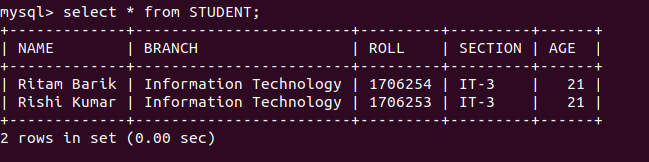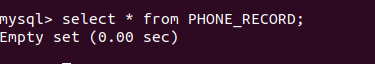# Python MySQL – Delete Query

Python Database API ( Application Program Interface ) is the Database interface for the standard Python. This standard is adhered to by most Python Database interfaces. There are various Database servers supported by Python Databases such as MySQL, GadFly, PostgreSQL, Microsoft SQL Server 2000, Informix, Interbase, Oracle, Sybase, etc. To connect with MySQL database server from Python, we need to import the `mysql.connector` interface.

Below is a program to connect with MySQL database `geeks`.

 `# importing required library  ` `import` `mysql.connector ` ` `  `# connecting to the database  ` `dataBase ``=` `mysql.connector.connect( ` `                     ``host ``=` `"localhost"``, ` `                     ``user ``=` `"user"``, ` `                     ``passwd ``=` `"pswrd"``, ` `                     ``database ``=` `"geeks"` `)  ` ` `  `# preparing a cursor object  ` `cursorObject ``=` `dataBase.cursor()  ` ` `  ` `  `# disconnecting from server ` `dataBase.close()  `

The above program illustrates the connection with the MySQL database `geeks` in which host-name is `localhost`, the username is `user` and password is `pswrd`.

### Deleting query from tables

After connecting with the database in MySQL we can create tables in it and can manipulate them.

Syntax Statement:

```DELETE FROM TABLE_NAME WHERE ATTRIBUTE_NAME = ATTRIBUTE_VALUE
```

Example 1: Below is a program to delete a query from the table in the database.

 `# importing required library  ` `import` `mysql.connector  ` ` `  `# connecting to the database  ` `dataBase ``=` `mysql.connector.connect( ` `                     ``host ``=` `"localhost"``, ` `                     ``user ``=` `"user"``, ` `                     ``passwd ``=` `"pswrd"``, ` `                     ``database ``=` `"geeks"` `)   ` ` `  `# preparing a cursor object  ` `cursorObject ``=` `dataBase.cursor()  ` ` `  `# creating table   ` `studentRecord ``=` `"""CREATE TABLE STUDENT (  ` `                   ``NAME  VARCHAR(20) NOT NULL,  ` `                   ``BRANCH VARCHAR(50),  ` `                   ``ROLL INT NOT NULL, ` `                   ``SECTION VARCHAR(5),  ` `                   ``AGE INT ` `                   ``)"""` ` `  `# table created ` `cursorObject.execute(studentRecord)   ` ` `  `# inserting data into the table ` `query ``=` `"INSERT INTO STUDENT (NAME, BRANCH, ROLL, SECTION, AGE) VALUES (% s, % s)"` ` `  `attrValues ``=` `(``"Rituraj Saha"``, ``"Information Technology"``, ``"1706256"``, ``"IT-3"``, ``"20"``) ` `cursorObject.execute(query, attrValues) ` ` `  `attrValues ``=` `(``"Ritam Barik"``, ``"Information Technology"``, ``"1706254"``, ``"IT-3"``, ``"21"``) ` `cursorObject.execute(query, attrValues) ` ` `  `attrValues ``=` `(``"Rishi Kumar"``, ``"Information Technology"``, ``"1706253"``, ``"IT-3"``, ``"21"``) ` `cursorObject.execute(query, attrValues) ` ` `  `# deleting query ` `query ``=` `"DELETE FROM STUDENT WHERE ROLL = 1706256"` `cursorObject.execute(query, attrValues) ` ` `  `dataBase.commit() ` ` `  `# disconnecting from server ` `dataBase.close() `

Output:In the above program, a table named `STUDENT` is created having attributes `NAME`, `BRANCH`, `ROLL`, `SECTION` and `AGE`. Multiple data is inserted into the `STUDENT` table and then a single query is deleted from the the table having the `ROLL` attribute value `1706256`.

Example 2: Let us look at another example for queries in a table.

 `# importing required library  ` `import` `mysql.connector ` ` `  `# connecting to the database  ` `dataBase ``=` `mysql.connector.connect( ` `                     ``host ``=` `"localhost"``, ` `                     ``user ``=` `"user"``, ` `                     ``passwd ``=` `"pswrd"``, ` `                     ``database ``=` `"geeks"` `)  ` ` `  `# preparing a cursor object  ` `cursorObject ``=` `dataBase.cursor()  ` ` `  `# drop table if it already exists  ` `cursorObject.execute(``"DROP TABLE IF EXISTS PHONE_RECORD"``) ` ` `  `# creating table   ` `phoneRecord ``=` `"""CREATE TABLE PHONE_RECORD (  ` `                   ``NAME  VARCHAR(20) NOT NULL,  ` `                   ``PHONE VARCHAR(10) NOT NULL ` `                   ``)"""` ` `  `# table created ` `cursorObject.execute(phoneRecord)   ` ` `  `# inserting data into the table ` `query ``=` `"INSERT INTO PHONE_RECORD (NAME, PHONE) VALUES (% s, % s)"` `attrValues ``=` `(``"Rituraj Saha"``, ``"9163089075"``) ` `cursorObject.execute(query, attrValues) ` ` `  `# deleting query ` `query ``=` `"DELETE FROM STUDENT WHERE NAME = 'Rituraj Saha'"` `cursorObject.execute(query) ` ` `  `dataBase.commit() ` ` `  `# disconnecting from server ` `dataBase.close() `

Output:In the above program, another table is created in the `geeks` database named `PHONE_RECORD` having attribute `NAME` and `PHONE`. Only one column is inserted into the table and then it is deleted using the `DELETE` statement.

My Personal Notes arrow_drop_upCheck out this Author's contributed articles.

If you like GeeksforGeeks and would like to contribute, you can also write an article using contribute.geeksforgeeks.org or mail your article to contribute@geeksforgeeks.org. See your article appearing on the GeeksforGeeks main page and help other Geeks.

Please Improve this article if you find anything incorrect by clicking on the "Improve Article" button below.

Article Tags :

Be the First to upvote.

Please write to us at contribute@geeksforgeeks.org to report any issue with the above content.